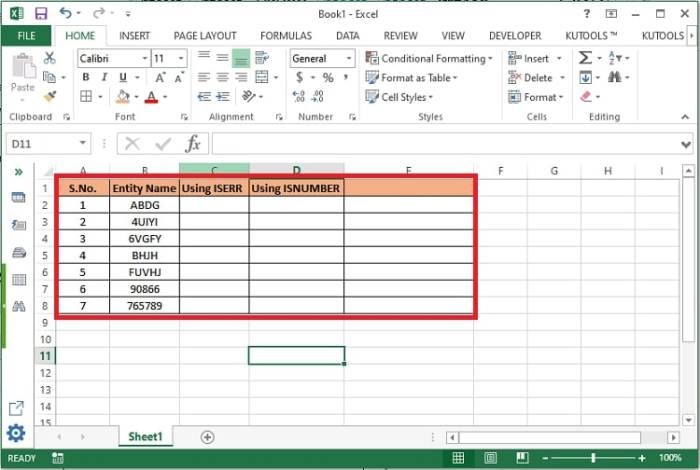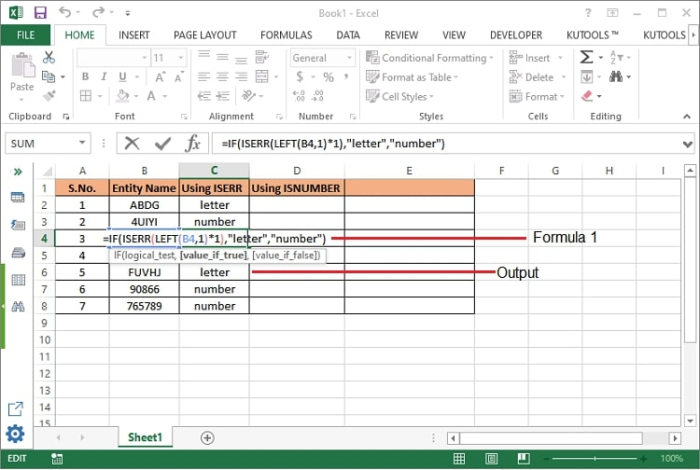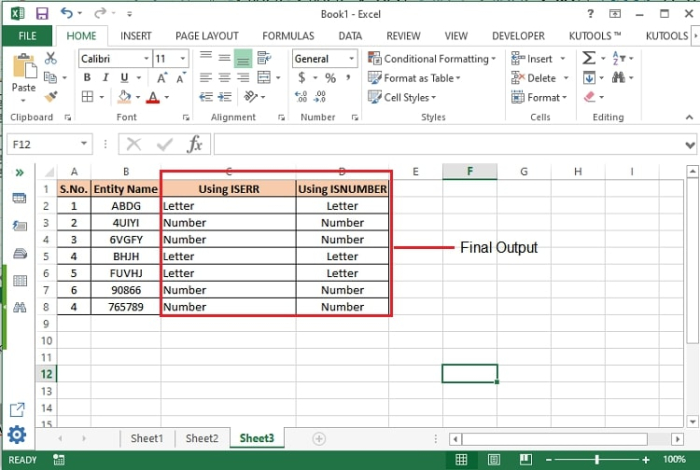# How to check if first character in a cell is a letter or number in Excel?

Excel has a bunch of formulas and data analysts use this application to prepare various reports and dashboards. To support that analysis this article will help an excel user to identify all the cells whose values either starts with a number or an alphabet from a collection of dataset. We will identify these cells using the combination of following formulas.

• IF function

• ISERR function

• Left function

## Check if first character in a cell is a number or a letter

The following formulas can be used to check if the first character of a cell is number or a letter.

• =IF(ISERR(LEFT(cell_location,1)*1),"Letter”, “Number")

In this formula, we are calculating the value of a cell’s left most character multiplied by 1. If the obtained value is not a number then it will return Letter else Number.

• =IF(ISNUMBER(VALUE(LEFT(cell_location, 1))),"Number", "Letter")

In this formula, we are calculating the value of left most character of a cell. If the returned value is a number, then it will return TRUE (by default) or as per the defined value (Number in our case).

Step 1 − A sample worksheet has been shown below with some sample data.Step 2 − Under the column C, the following formula has been used to get the desired result. Enter the formula in a cell and press enter.

=IF(ISERR(LEFT(B2,1)*1),"Letter”, “Number")Step 3 − Now drag the cell to copy the formula in other cells also to view the output against all cells. Enter the formula in a cell and press enter.

Step 4 − Under the Column D, the following formula has been used to get the desired result.

=IF(ISNUMBER(VALUE(LEFT(B2, 1))),"Number", "Letter")Step 5 − Now drag the cell to copy the formula in other cells also to view the output against all cellsFormula Syntax Description

Argument Description
IF(logical_test, {value_if_true},{value_if_false}
• Logical_test specifies the condition basis which the data needs to be rendered.

• Value_if_true specifies the value that shall be returned if the condition satisfies.

• Value_if_false specifies the value that shall be returned if the condition does not satisfy.

LEFT(text, [num_chars])
• Text specifies the cell address whose value to be returned or scanned from left.

• num_chars specifies the number of characters from left that need to be returned/scanned.

ISERR(value)
• The ISERR function returns TRUE for any error type except the #N/A error. For example, #REF!, #DIV?0!, #VALUE!, #NAME? etc. ISERR function can be used with the IF function to test for an error, display a custom message, or for a different calculation if found.

• (value) points to the value of the selected cell where the error to be identified.

## Conclusion

The start or end character of a cell value can be identified using many other combinations of excel functions. However, these the most used functions for the same. The same functions can be used to identify the cell ending with a number or letter by replacing LEFT from RIGHT.

• =IF(ISERR(RIGHT(cell_location,1)*1),"Letter”, “Number")

• =IF (ISNUMBER(VALUE(RIGHT(cell_location, 1))),"Number", "Letter")